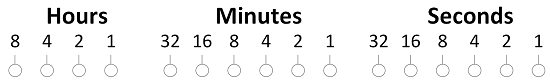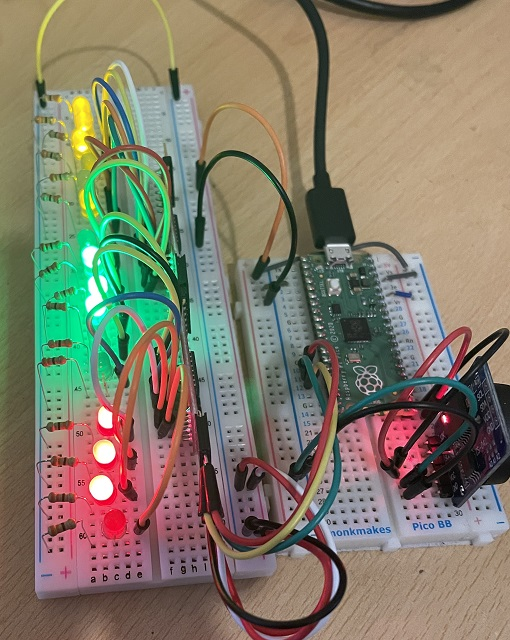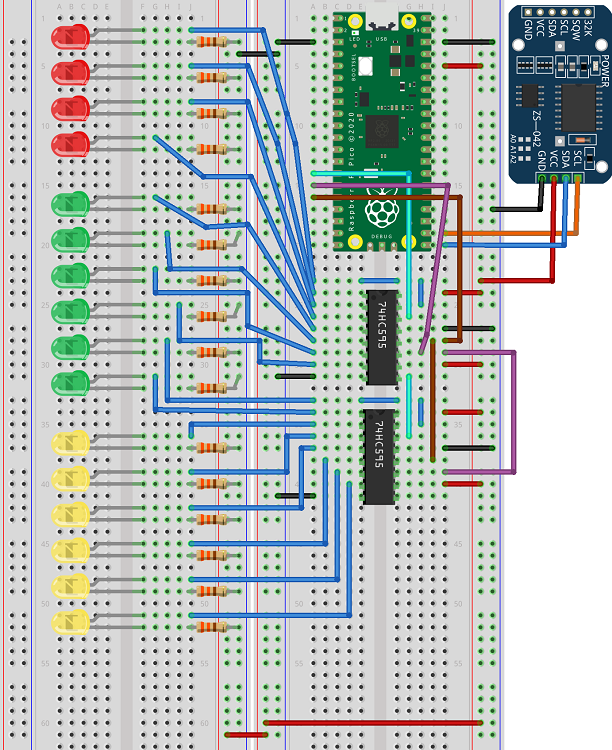# Raspberry Pi Pico Binary Clock

Making a binary clock has become an obligatory project for me when I get a new microcontroller. This one uses two 74HC595 shift registers chained together and 16 LEDs. With 16 LEDs, the time breaks down as follows,That means that we go with 12 hour time.

In the version I connected to the Pico, I used red LEDs for the hours, green for minutes and yellow for seconds. I cheated a little by using Sparkfun breakout boards for the shift registers. A mixture of male and female right angled headers allows you to connect them together.Using normal integrated circuits, you can do this on some breadboards. Here is the Fritzing version of that. Imagine the whole thing rotated 90 degrees anticlockwise.When you chain together more than one shift register, you follow a similar protocol to using just one. The difference is that you toggle the latch after you have clocked in all 16 bits. You need to do some bitshifting to get the whole time into a 16 bit pattern. I am using a a DS3231 RTC and the library that I wrote.

```from machine import Pin
from time import sleep
from ds3231 import rtc

data = Pin(10, Pin.OUT)
clock = Pin(11, Pin.OUT)
latch = Pin(12, Pin.OUT)

# subroutine to shift binary data out, n = 2 bytes
def shift_out(n, d, c, l):
d.value(0)
c.value(0)
l.value(0)
bits = [n >> i & 1 for i in range(16)]
for i in range(16):
d.value(bits[i])
c.value(1)
c.value(0)
l.value(1)
l.value(0)

myclock = rtc(16, 17)

# s,m,h,w,dd,mm,yy
#myclock.set_time(0,2,7,1,14,2,2022)

while True:
t = myclock.get_time()
hr = t
if hr>12: hr -= 12
mins = t
secs = t
lbyte = (hr<<4) + (mins>>2)
rbyte = ((mins&3)<<6)+secs
tm = (lbyte<<8) + rbyte
shift_out(tm, data, clock, latch)
print(hr,mins,secs)
sleep(1)
```